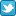Warning: this one is for the really, really, super duper, geeks.

Ok, now that nobody's left I can talk to myself...

Anyway, TinyP2P is a peer-to-peer file sharing application, written in fifteen lines of code (written in Python).

Every line has 80 characters or less!

Quite amazing.

And if you've read this far then you probably want to see these 15 lines so here goes:

# tinyp2p.py 1.0 (documentation at http://freedom-to-tinker.com/tinyp2p.html)
import sys, os, SimpleXMLRPCServer, xmlrpclib, re, hmac # (C) 2004, E.W. Felten
ar,pw,res = (sys.argv,lambda u:hmac.new(sys.argv,u).hexdigest(),re.search)
pxy,xs = (xmlrpclib.ServerProxy,SimpleXMLRPCServer.SimpleXMLRPCServer)
def ls(p=""):return filter(lambda n:(p=="")or res(p,n),os.listdir(os.getcwd()))
myU,prs,srv = ("http://"+ar+":"+ar, ar[5:],lambda x:x.serve_forever())
def pr(x=[]): return ([(y in prs) or prs.append(y) for y in x] or 1) and prs
def c(n): return ((lambda f: (f.read(), f.close()))(file(n)))
f=lambda p,n,a:(p==pw(myU))and(((n==0)and pr(a))or((n==1)and [ls(a)])or c(a))
def aug(u): return ((u==myU) and pr()) or pr(pxy(u).f(pw(u),0,pr([myU])))
pr() and [aug(s) for s in aug(pr())]
(lambda sv:sv.register_function(f,"f") or srv(sv))(xs((ar,int(ar))))
for url in pxy(ar).f(pw(ar),0,[]):
for fn in filter(lambda n:not n in ls(), (pxy(url).f(pw(url),1,ar))):
(lambda fi:fi.write(pxy(url).f(pw(url),2,fn)) or fi.close())(file(fn,"wc"))

Update:

Somebody wrote an even shorter version in Perl.

This one is only 9 lines and doesn't use any external libraries!!

Check it out: http://ansuz.sooke.bc.ca/software/molester/

\$p=shift;\$a=shift;i(shift);socket S,2,1,6;bind S,&a(\$a);listen
S,5;\$/=undef;while(@ARGV&&(\$_="\$p \$a f".shift)||accept(C,S)&&(\$_=)&&close
C){m!^(.*?) (.*?) ([e-i])([^/]*)/!s&&\$1 eq\$p&&&\$3(\$2,\$4,\$');}sub e{open
F,'>',\$_;print F \$_;close F}sub f{&s(\$_,@_)for keys %k}sub
g{open(F,'<',\$_)&&&s(\$_,\$a,"e\$_",);close F}sub
h{&s(\$_,\$_,'i')for keys %k}sub i{\$k{\$_}=1}sub
a{\$_=~/:/;pack'CxnC4x8',2,\$',split'\.',\$`}sub
s{socket X,2,1,6;\$w=shift;if(connect X,&a(\$w)){print X
"\$p \$_ \$_/\$_";close X}else{undef \$k{\$p}}}

Now you see why I love Perl...Tweet this postShare on Facebook